### Keyword POINTS

Points for the plotting of molecular fields (4.10.3) and starting points for the critical point search (4.10.4) can be specified with this keyword.
Options:
BINARY / ASCII / POLYGON / READ
 BINARY The plot point coordinates and connectivities are read from the external binary file LAT.bin. This is the default. ASCII The plot point coordinates and connectivities are read from the external ascii file LAT.asc. POLYGON Starting points for the critical point search are generated by the polygon algorithm. READ The plot point coordinates are read from the file deMon.inp.
Description:
The option BINARY causes coordinates and connectivities to be read from the file LAT.bin. Usually this file has been produced by a previous run which calculated isosurfaces or geometrical surfaces (see Sections 4.10.5 and 4.10.6). In this way, molecular fields can be plotted on isosurfaces. Exampleon pageof the deMon2k tutorial describes the plotting of the electrostatic potential on the isodensity surface of benzene. The LAT.bin file and the corresponding plot output can be visualized with VU . With the ASCII option, plot point coordinates, and optionally, connectivities, can be read from the external ascii file LAT.asc. The file format is:

```
1814
.312196E+00    -.729474E+00     .105260E+01
.243158E+00    -.765796E+00     .105260E+01
.243158E+00    -.729474E+00     .917119E+00
```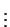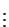```
1          2          3
4          5          6
6          7          8
```The first integer (here 1814) denotes the number of points. For each point, a line is then given with its coordinates (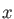,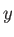and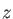). The integer triples after the coordinate specifications are the connectivities. This part of the input is optional. The LAT.asc file can be generated easily from the deMon.lat file (see 4.10.5) and used directly for visualization with VU.

With the POLYGON option, starting points for the critical point search are generated automatically. In the case of the electronic density, this algorithm usually generates a sufficient set of starting points to find all critical points. This is not the case for the critical points of other molecular fields. The READ option can be used to read point coordinates from the input file deMon.inp. The coordinates are given in free format in the keyword body of POINTS, one input line for each point.